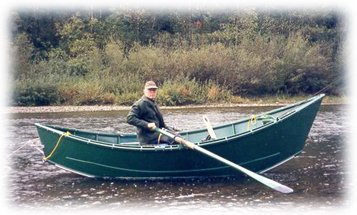# River and Boat riddle

723.6K Views

John and Jacob are on the two opposite banks of a river. They both have a rowing boat.

They both start at the same time towards the opposite bank. They pass each other at 180 meters from the bank where john departed. When reaching the opposite bank, they both take a rest for the same amount of time before they return. On the way back, they pass each other at 100 meters from the bank from where John returned.

John and Jacob both row with a constant speed, but Jacob rows faster.

How wide is the river?440 metersI hate it when people only post the answer and not how they came to this answer.
Some people come here to learn how to solve this kind of problems.

I know that there are people on this site that don’t like it when someone else give the same answer without upvoting there answer so I upvoted your answer.

We want to calculate the width of the river X.
Jacob rows with speed v1 and John with v2.
The first time they pass eachother (at time t1) the total distance they rowed is X
And John rowed 180m

v1*t1+v2*t1 = X
v2*t1 = 180
t1 = 180/v2
180*v1/v2 + 180 = X
v1 = v2(X – 180)/180

The time they rest is not important so we calcutate it as if they didn’t rest.
The second time they pass eachother (at time t2) the total distance they rowed is 3X.
And John rowed X plus 100m

v1*t2+v2*t2 = 3X
v2*t2 = X+100
t2 = (X+100)/v2
(X+100)*v1/v2 + X+100 = 3X
v1 = v2(2X-100)/(X+100)

v2(X – 180)/180 = v2(2X-100)/(X+100)
(X – 180)/180 = (2X-100)/(X+100)
(X – 180)*(X+100) = 180*(2X-100)
X*X – 80X – 18000 = 360X -18000
X*X – 80X = 360X
X = 440

on 14th November 2018.well my typing speed is not good

on 15th November 2018.